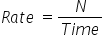Mathematics
Easy

Question

# Paul can eat 5 hot dogs in 10 minutes. _________ minutes are taken by him to eat 30 hot dogs

## 60905050Hint:

## The correct answer is: 60

### Let the number of hot dogs be denoted by N.So the given values of number of hot dogs and time are:N = 5Time = 10 minutesUsing this data, we will find the rate. We will find the rate by taking the ratio of number of hot dogs to the time. As rate is constant, we will use this to find the time required by Paul to eat 30 hot dogs.Now using the value of rate we will find the time requiredWe will rearrange the expression for time.So, Paul takes 60 minutes to eat 30 hot dogs.

The other method is to take the ratios and directly equate them. As the rate is constant, so the ratios will be constant. So we will consider the quantity we have to find as x and then equate the ratios. It will be a shortcut.

### Related Questions to study#### With Turito Foundation.#### Get an Expert Advice From Turito.